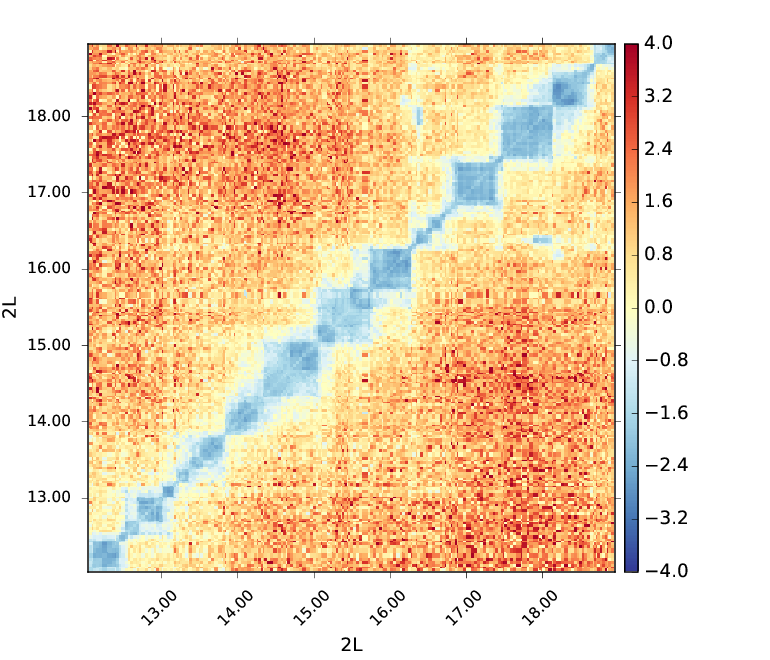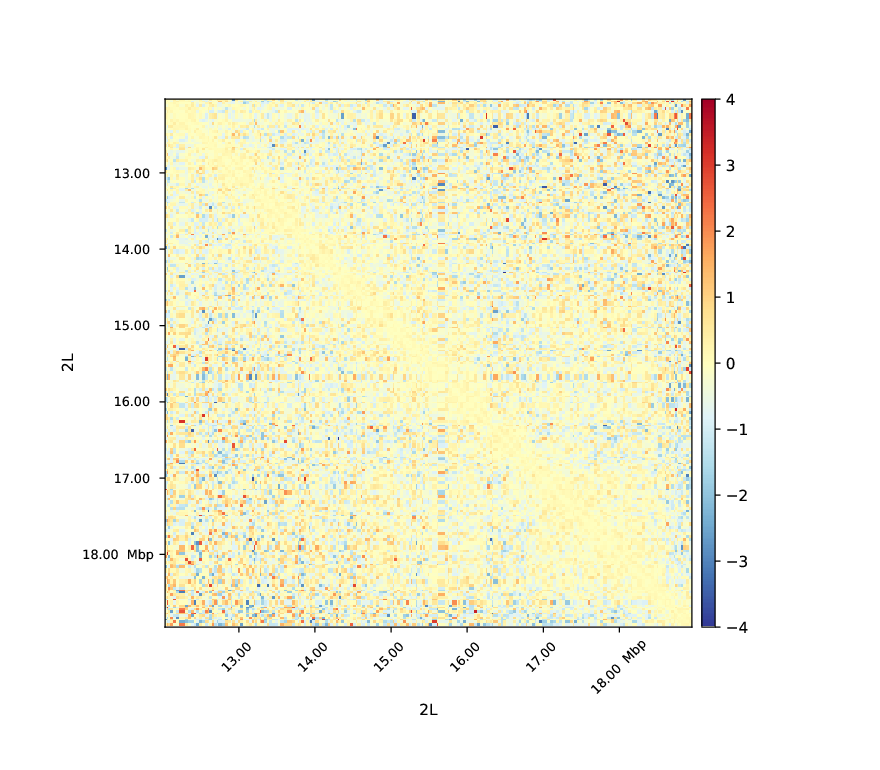# hicCompareMatrices¶

## Background¶

This tool is useful to compare two matrices in .h5 format by applying operations like difference, ratio or log2ratio after normalization. This can be used to determine the effect of a mutation compared to wild-type samples on contact enrichment, or to see TAD structure modifications near differentially expressed genes between two conditions when followed by hicPlotMatrix. It can also be used to compare two biological replicates.

## Description¶

Takes two matrices as input, normalizes them and applies the given operation. To normalize the matrices each element is divided by the sum of the matrix.

usage: hicCompareMatrices --matrices matrix.h5 matrix.h5 --outFileName
OUTFILENAME [--operation {diff,ratio,log2ratio}]
[--help] [--version]


### Required arguments¶

--matrices, -m

Name of the matrices in .h5 format to use, separated by a space.

--outFileName, -o

File name to save the resulting matrix. The output is also a .h5 file.

### Optional arguments¶

--operation

Possible choices: diff, ratio, log2ratio

Operation to apply to the matrices (Default: “log2ratio”).

Default: “log2ratio”

--version

show program’s version number and exit

## Usage example¶

hicCompareMatrices is usually perfomed on corrected matrices (hicCorrectMatrix) with bins merged (hicMergeMatrixBins) depending on the downstream analyses to perform. Here is an example of a log2ratio comparison between M1BP Knockdown and GST cells in Drosophila melanogaster on corrected matrices with 50 bins merged (about 30kb bins).

hicCompareMatrices -m \
M1BP_KD_merge_m50_corrected.h5 \
GST_merge_rf_m50_corrected.h5 \
--operation log2ratio -o m1bp_over_gst_log2_m50.h5


This code outputs a matrix containing the normalized log2ratio values of M1BP_KD_merge_m50_corrected.h5 over GST_merge_rf_m50_corrected.h5. We can then display this matrix using hicPlotMatrix.

hicPlotMatrix -m \
m1bp_over_gst_log2_m50.h5 \